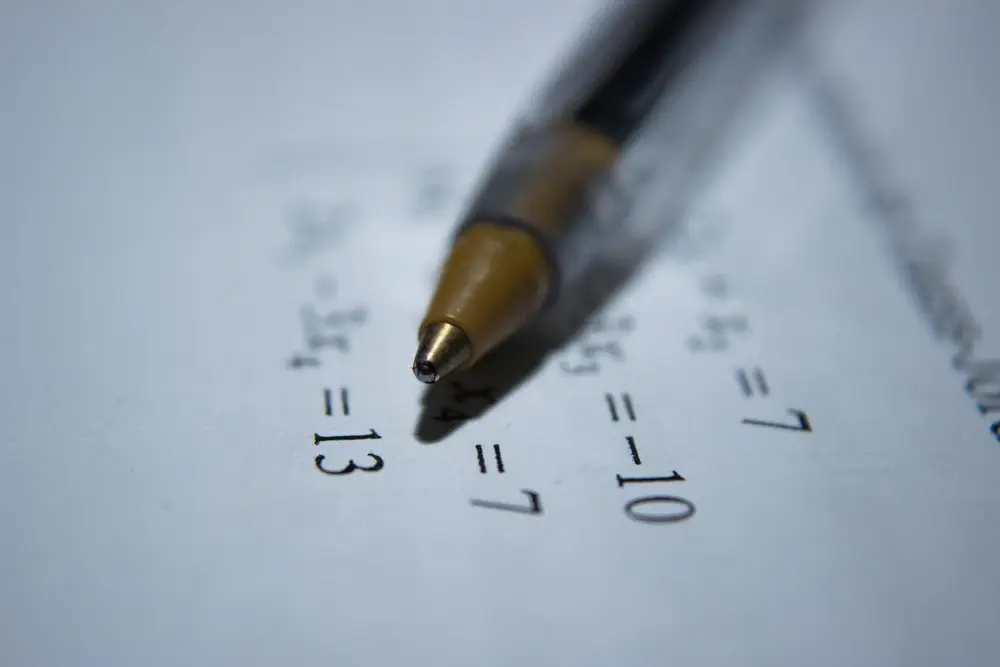# Proportion

Two quantities which vary together are said to have proportionality with each other. Proportionality between two values is shown using the symbol: which means is proportional to. There are several types of proportionality.

Direct Proportion

•  b means a is directly proportional to b. This means as a changes so does b. The connection involves a constant which can be seen as a = kb where k is the constant of proportionality. When a and b are plotted on a graph it gives a straight line which passes through (0,0) and has a gradient of k.

Inverse Proportion

• 1/b means a is inversely proportional to b. This means as a increases b decreases. This connection involves a division which can be seen as a = k/b where k is the constant of proportionality. When a and b are plotted on a graph it should give a reciprocal graph.

These are the basics of proportionality. There are many more types of proportionality and many topics connected to it. You should do more in depth revision on this subject.

Algebra

Algebra

Algebra

Algebra

Algebra

Algebra